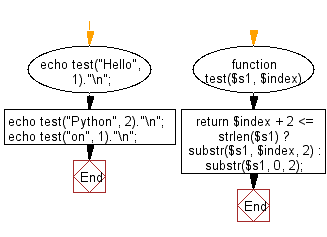﻿ PHP Exercises: Create a new string of length 2 starting at the given index of a given string - w3resource# PHP Exercises: Create a new string of length 2 starting at the given index of a given string

## PHP Basic Algorithm: Exercise-73 with Solution

Write a PHP program to create a new string of length 2 starting at the given index of a given string.

Sample Solution:

PHP Code :

``````<?php
function test(\$s1, \$index)
{

return \$index + 2 <= strlen(\$s1) ? substr(\$s1, \$index, 2) : substr(\$s1, 0, 2);

}

echo test("Hello", 1)."\n";
echo test("Python", 2)."\n";
echo test("on", 1)."\n";
``````

Sample Output:

```el
th
on
```

Flowchart:PHP Code Editor:

What is the difficulty level of this exercise?

﻿

## PHP: Tips of the Day

\$_REQUEST: This SuperGlobal Variable is used to collect data submitted by a HTML Form

Example:

This code save in a php file and run in the browser.

```<!DOCTYPE html>
<html>
<body>

<form method="post" action="<?php echo \$_SERVER['PHP_SELF'];?>">
NAME: <input type="text" name="user">
<button type="submit">SUBMIT</button>
</form>
<?php
if (\$_SERVER["REQUEST_METHOD"] == "POST") {
\$name = htmlspecialchars(\$_REQUEST['user']);
if(empty(\$name)){
echo "Name is empty";
} else {
echo \$name;
}
}
?>
</body>
</html>
```

Output:

```Owen
```